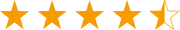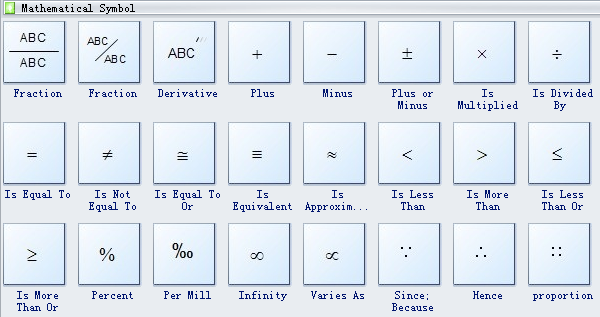A versatile cross-platform mind mapping tool.Get from App StoreA versatile cross-platform mind mapping tool.Get from App Store
edraw-max-horizontal
Product Tour
Guide
What's New
EdrawMax Online
TRY IT FREE

# Mathematics Diagram

> Basic Diagram Knowledge > Mathematics Diagram
Posted by James Freeman | 12/23/2019
Creating mathematics diagram with free templates and examples. Mathematics diagram has never been easier.

### Mathematics Diagram

Mathematics is a study of subjects such as quantity, numbers, structure, space and change. Mathematics is used all around the world as an important tool in many areas, including natural science, engineering, medicine, finance and the social science, etc.
With Edraw software, you can easily draw all kinds of Greek letters, mathematical formulas, and geometric figures including any point, line, segment, ray, angle, polygon, curve, region, plane, surface, solid, etc.

### Mathematics Diagram Software

With Edraw's Mathematics template, you can easily create educational math illustrations with the shapes of plane and solid geometric figures. Edraw allows you both hand-draw and drag-and-drop pre-made shapes, which makes drawing incredibly easy. You can specify the exact locations of curves and shapes when you need better precision. It's absolutely the most convenient and easy drawing tool you ever found.

### Mathematics Diagram Symbols

There are many mathematical symbols in Edraw software, fraction, derivative, plus, minus, plus or minus, is multiplied, is divided by, is equal to, is not equal to, is equal to or, is equivalent to, is approximately equal to, is less than, is more than, etc.